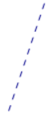# LineBrowse the sample

The .NET Multi-platform App UI (.NET MAUI) Line class derives from the Shape class, and can be used to draw lines. For information on the properties that the Line class inherits from the Shape class, see Shapes.

Line defines the following properties:

• X1, of type double, indicates the x-coordinate of the start point of the line. The default value of this property is 0.0.
• Y1, of type double, indicates the y-coordinate of the start point of the line. The default value of this property is 0.0.
• X2, of type double, indicates the x-coordinate of the end point of the line. The default value of this property is 0.0.
• Y2, of type double, indicates the y-coordinate of the end point of the line. The default value of this property is 0.0.

These properties are backed by BindableProperty objects, which means that they can be targets of data bindings, and styled.

For information about controlling how line ends are drawn, see Control line ends.

## Create a Line

To draw a line, create a Line object and set its `X1` and `Y1` properties to its start point, and its `X2` and `Y2` properties to its end point. In addition, set its Stroke property to a Brush-derived object because a line without a stroke is invisible. For more information about Brush objects, see Brushes.

Note

Setting the Fill property of a Line has no effect, because a line has no interior.

The following XAML example shows how to draw a line:

``````<Line X1="40"
Y1="0"
X2="0"
Y2="120"
Stroke="Red" />
``````

In this example, a red diagonal line is drawn from (40,0) to (0,120):Because the X1, Y1, X2, and Y2 properties have default values of 0, it's possible to draw some lines with minimal syntax:

``````<Line Stroke="Red"
X2="200" />
``````

In this example, a horizontal line that's 200 device-independent units long is defined. Because the other properties are 0 by default, a line is drawn from (0,0) to (200,0).

The following XAML example shows how to draw a dashed line:

``````<Line X1="40"
Y1="0"
X2="0"
Y2="120"
Stroke="DarkBlue"
StrokeDashArray="1,1"
StrokeDashOffset="6" />
``````

In this example, a dark blue dashed diagonal line is drawn from (40,0) to (0,120):